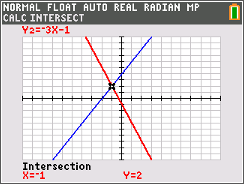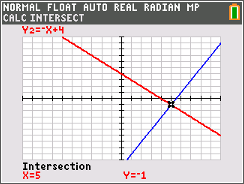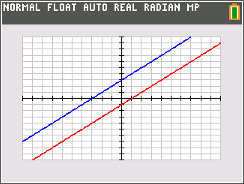# Activities

••• ##### Subject Area

• Math: Algebra II: Systems of Linear Equations and Inequalities

• ##### Author9-12

45 Minutes

• ##### Device
• TI-84 Plus
• TI-84 Plus Silver Edition
•TI-84 Plus C Silver Edition
•TI-84 Plus CE
• ##### Report an Issue

Intersecting the Solution#### Activity Overview

Students will learn to solve systems of equations graphically and understand the relationship between the algebraic and graphical solutions.

#### Key Steps

•In the first part of the activity, students will solve a system of equations algebraically and then solve it graphically using their graphing calculator. In this comparison, students are to determine how the point of intersection relates to the algebraic solution.

•Then, students will modify existing equations to change the slope and the y-intercept of two lines. Their goal is to change the lines so that they both intersect a given point on the graph. Students should be encouraged to find more than one system of equations that work.

•The last part of the activity can be used as an extension if time is limited. Students will change the two lines on the graph so that the system has no solutions and then has infinite many solutions. Discussion questions for all parts of the activity are given on the teacher document.